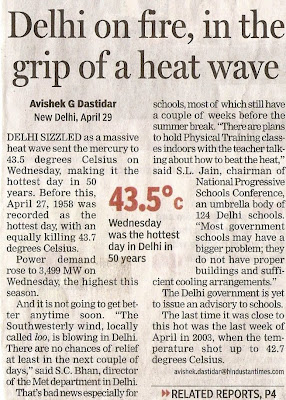## Thursday, April 30, 2009

### Mausam garam hai, nah? (The weather is hot, no? More or less.)

Holy mackerel it's hot here. Now, let's see. To convert Celsius to Fahrenheit, first you divide the Celsius number by the square root of negative one, then multiply by two times the square root of two then add the last number of pi, then multiply by the circumference, right? What does that equal? Oh yeah, one billion degrees. Of all the things I will miss about India, and there are many, I assure you (Dal Makhni anyone?), I will not miss the seventeen crore lakh degree weather we are experiencing right now.#### 1 comment:

*~NiTiN~* said...

Well.....

Celsius to Fahrenheit (°C × 9/5) + 32 = °F

Fahrenheit to Celsius (°F - 32) x 5/9 = °C

It isn't that difficult!!